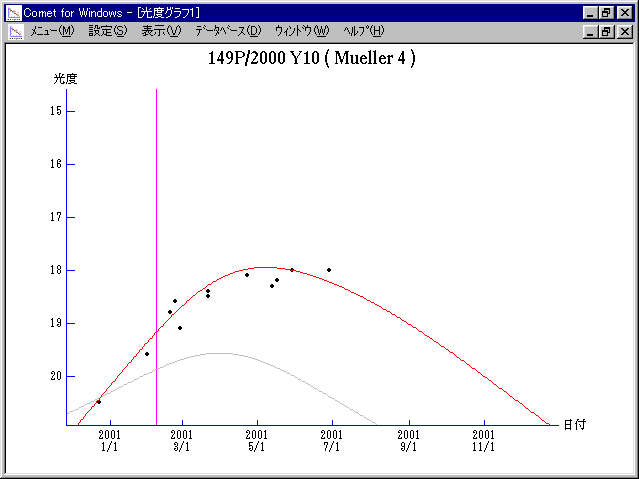# \$B%_%e%i!

149P/Mueller 4 (2001)###\$B%W%m%U%#!<%k(B

 \$BId9f(B 149P/2000 Y10 \$B8!=PF|(B 2000\$BG/(B12\$B7n(B22\$BF|(B \$BH/8+8wEY(B 20.5\$BEy(B \$BH/8+ T. Oribe (Saji Observatory)

###\$B50F;MWAG(B

```   The following improved orbital elements by Kenji Muraoka, are
from 57 observations 1992 Apr. 9 to 2001 Mar. 25, perturbations
by 9 Plantes, Moon and 5 minor planets were taken into account.
The mean residual is +/- 0.78 arc seconds.

Epoch  =  2001 Feb. 20.0  TT       JDT = 2451960.5
T  =  2001 Feb.  7.82229       +/- 0.01064 (m.e.) TT
Peri. =   43.59960                +/- 0.00297
Node  =  145.36886                +/- 0.00027   (2000.0)
Incl. =   29.74897                +/- 0.00012
q  =    2.6466976              +/- 0.0000106 AU
e  =    0.3885979              +/- 0.0000025
a  =    4.3288983              +/- 0.0000057 AU
n  =    0.10943040             +/- 0.00000022
P  =    9.007                  +/- 0.0000177  years
```

###\$B@1?^(B###\$B8wEYJQ2=(B

```        m1 = -2.3 + 5 log\$B&\$(B + 44 log r(t - 150)

\$B"((B \$B3%?'\$N6J@~\$O!"(Bm1 = 14.0 + 5 log\$B&\$(B + 10.0 log r \$B\$N8wEY6J@~\$G\$9!#(B
```##### \$B50F;MWAG\$OB<2,7r<#;a\$N7W;;\$K\$h\$k\$b\$N\$G\$9!#(B \$B@1?^\$O(B StellaNavigator Ver.2.0 for Windows (\$B%"%9%H%m%"!<%D(B \$BJTCx(B / \$B%"%9%-!<=PHG6I4)(B) \$B\$G:n@.\$7\$?\$b\$N\$G\$9!#(B \$B8wEY%0%i%U\$O(BComet for Windows\$B\$G:n@.\$7\$?\$b\$N\$G\$9!#(B